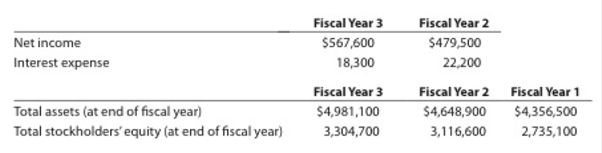# Profitability ratios Ralph Lauren Corporation sells apparel through company-owned retail stores. Recent financial information for Ralph Lauren follows (in thousands): Assume that the apparel industry average return on total assets is 8.0% and the average return on stockholders’ equity is 10.0% for the year ended April 2, Year 3 a. Determine the return on total assets for Ralph Lauren for fiscal Years 2 and 3 Round percentages to one decimal place. b. Determine the return on stockholders’ equity for Ralph Lauren for fiscal Years 2 and 3. Round percentages to one decimal place. c. Evaluate the two-year trend for the profitability ratios determined in (a) and (b). d. Evaluate Ralph Lauren’s profit performance relative to the industry.### Accounting

27th Edition
WARREN + 5 others
Publisher: Cengage Learning,
ISBN: 9781337272094### Accounting

27th Edition
WARREN + 5 others
Publisher: Cengage Learning,
ISBN: 9781337272094

#### Solutions

Chapter
Section
Chapter 17, Problem 17.18EX
Textbook Problem

## Profitability ratiosRalph Lauren Corporation sells apparel through company-owned retail stores. Recent financial information for Ralph Lauren follows (in thousands):Assume that the apparel industry average return on total assets is 8.0% and the average return on stockholders’ equity is 10.0% for the year ended April 2, Year 3a. Determine the return on total assets for Ralph Lauren for fiscal Years 2 and 3 Round percentages to one decimal place.b. Determine the return on stockholders’ equity for Ralph Lauren for fiscal Years 2 and 3. Round percentages to one decimal place.c. Evaluate the two-year trend for the profitability ratios determined in (a) and (b).d. Evaluate Ralph Lauren’s profit performance relative to the industry.

Expert Solution

a)

To determine

Financial Ratios: Financial ratios are the metrics used to evaluate the liquidity, capabilities, profitability, and overall performance of a company.

To determine: Return on total assets for RL for fiscal years 2 and 3

Given info: Selected items from the financial statements for fiscal years 1, 2, and 3.

### Explanation of Solution

Return on assets determines the particular company’s overall earning power. It is determined by dividing sum of net income and interest expense and average total assets.

Formula:

Rate of return on assets=Netincome + Interest expenseAverage total assets

Working notes for determining average total assets are as follows:

Average total assets for fiscal year 3

Average total assets for fiscal year 3}=Opening assets + Closing assets 2=\$4,981

Expert Solution

b)

To determine

To determine: Determine return on stockholders’ equity.

Expert Solution

c)

To determine

To evaluate: Two-year trend for the profitability ratios.

Expert Solution

d)

To determine

To evaluate: RL’s profit performance relative to the industry

### Want to see the full answer?

Check out a sample textbook solution.See solution

### Want to see this answer and more?

Bartleby provides explanations to thousands of textbook problems written by our experts, many with advanced degrees!

See solution